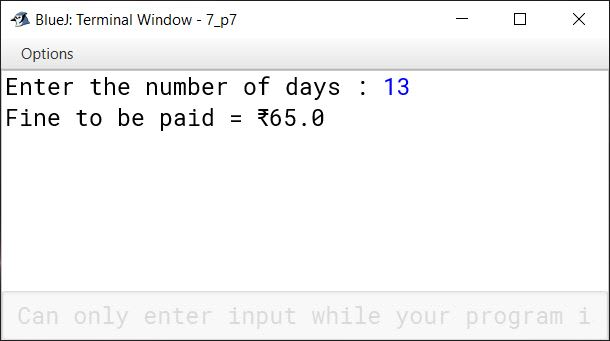# Input using Scanner Class

## Choose the correct option

#### Question 1

For which of the following purposes, the Scanner class is used?

1. To display a value
2. To find square root of a number
3. To input a value
4. To find maximum of two numbers

To input a value

Reason — The Scanner class allows the user to input values of different data types.

#### Question 2

Which of the following statements is true for nextDouble( )?

1. It accepts an integer type value
2. It accepts a double type value
3. It accepts a float type value
4. It accepts a long type value

It accepts a double type value

Reason — nextDouble( ) method receives a double type value and stores it in a double type variable.

#### Question 3

Which of the following does not find the square of a number?

1. Math.pow(a,2)
2. a*a
3. Math.sqrt(a,2)
4. a**2

Math.sqrt(a,2) and a**2

Reason — Math.sqrt(a,2) finds square root of the number. a**2 is an invalid expression in Java. It will cause a syntax error.

#### Question 4

What type of value is returned by Math.sqrt( )?

1. int
2. float
3. double
4. long

double

Reason — Return type of Math.sqrt( ) is double.

#### Question 5

Which of the following functions will find the square root of a number 'a'?

1. sqrt(a)
2. Math.sqrt(a)
3. Squareroot(a)
4. √a

Math.sqrt(a)

Reason — Math.sqrt() function is used to find the square root of a positive number.

#### Question 6

Which of the following package will you import to use Scanner class?

1. java_util;
2. Scanner.class;
3. java.util;
4. java.scanner.util;

java.util;

Reason — The Scanner class is available in the system package java.util. One must import java.util package to avail the facilities of the Scanner class.

## Fill in the blanks

#### Question 1

A class of JDK 1.5 version used to accept the values from the keyboard is known as Scanner.

#### Question 2

java.util package is necessary to be imported in order to use Scanner class.

#### Question 3

A function of Scanner class used to input integer numbers is nextInt().

#### Question 4

The function Math.sqrt( ) will result in square root of a number.

#### Question 5

System.in receives the input from the keyboard for the Scanner object.

#### Question 6

nextDouble() is a function to input a double type value.

## State True or False

#### Question 1

The Scanner class is a useful package of JDK 1.5.
False

#### Question 2

Integers can even be input without using the Scanner object.
True

#### Question 3

The word 'new' is a keyword used for dynamic allocation.
True

#### Question 4

The Math.max( ) function always returns double type value.
False

#### Question 5

The nextFloat( ) can also accept an integer number.
True

#### Question 6

The Math.min( ) can also find the minimum of three numbers.
False

## Predict the output for the following snippets

#### Question 1

```int x = 1,y = 1;
if(n > 0)
{
x = x + 1;
y = y + 1;
}
System.out.println(x + " , " + y);```

What will be the values of x and y, if the value of n is given as:
(i) 1
(ii) 0 ?

##### Output

(i) When n is 1:
x is 2 and y is 2

(ii) When n is 0:
x is 1 and y is 1

##### Explanation

When n is 1, `if (n>0)` is true. So the statements `x=x+1;` and `y=y+1;` are executed making the values of x and y as 2.

When n is 0, `if (n>0)` is false. Statements `x=x+1;` and `y=y+1;` are not executed so values of x and y remain 1.

#### Question 2

```int a = 20, b=15;
if ( a > 10 )
{
a = a++;
b++;
}
System.out.println( a + ","	+ b);```

What will be the values of a and b when executed?

##### Output
``````20,16
``````
##### Explanation

The value of `a` is 20 and `b` is 16. The condition `(a > 10)` is true, so the execution enters the if block. The statement `a = a++;` increments the value of `a` by 1 after the assignment. So `a` remains unchanged as we are assigning the original value of `a` (which is 20) back to `a`. The value of `b` is incremented by 1 so `b` becomes 16.

## Case-Study Based Question

#### Question 1

Mohit wanted to design a program using scanner class. Due to some confusion, he could not complete the program and has left some places marked with (i), (ii), (iii) and (iv) to be filled with the keywords/expression, as given below:

``````(i) java.util;
class Sample
{
public static void main(String args[])
{
(ii) num;
Scanner inp = (iii) Scanner(System.in);
System.out.println("Enter a number:");
num=(iv);
if(n % 2 == 0):
System.out.println("Even number");
else:
System.out.println("Odd number");
}
}
``````

Help him to complete the program by answering the following questions:

(a) What keyword/expression will be filled in place of (i)?

(b) What keyword/expression will be filled in place of (ii)?

(c) What keyword/expression will be filled in place of (iii)?

(d) What keyword/expression will be filled in place of (iv)?

(a) import

(b) int

(c) new

(d) inp.nextInt();

## Explain the following Java functions with an example

#### Question 1

Math.pow( )

In Java, Math.pow( ) function returns the value of the first argument raised to the power of the second argument.

Syntax

``````<Return data type> <variable> = Function name (argument 1, argument 2);

double <variable> = Math.pow(arg1, arg2);
``````

For example, consider the statement

`double d = Math.pow(3, 2);`

It will raise 3 to the power of 2 (3 * 3) and return a double type value for d as 9.0.

#### Question 2

Math.sqrt( )

This function is used to find the square root of a positive number. It will always return a double type value.

Syntax

``````<Return data type><variable> = Function name (Positive number);

double <variable> = Math.sqrt(arg);
``````

For example, consider the statement

`double n = Math.sqrt(25);`

It will return a double type value for n as 5.0.

#### Question 3

Math.min( )

This function is used to return the minimum of two numbers. It returns an int value if both of its arguments are int otherwise it returns a double value.

Syntax

``````<Return data type><variable> = Function name (argument 1, argument 2);

double <variable> = Math.min(doubleArg1, doubleArg2);

int <variable> = Math.min(intArg1, intArg2);
``````

For example, consider the statement

`int n = Math.min(91, 89);`

It will return the min value for n as 89.

The statement

`double d = Math.min(52, 89.7);`

will return a double type value for d as 52.0

#### Question 1

What do you mean by the Scanner class?

The Scanner class is one of the ways to input data values into the computer using Java language. It is defined inside the java.util package. It provides various next methods to accept values of different data types as input from the user through the keyboard.

#### Question 2

What is the need to input a value to the computer?

Some of the reasons why a program might need to accept input values from a user are:

1. To allow the program to perform calculations or processes using data provided by the user.
2. To enable the program to make decisions or take actions based on user input.
3. To allow the program to interact with the user and receive commands or instructions.
4. To change the behaviour of the program making it more specific to the user's needs based on the input data.

#### Question 3

Write syntax along with an example to create a Scanner object.

Syntax to create a Scanner object is:

`Scanner sc = new Scanner (System.in);`

Here, `sc` is an object of Scanner type.

For example, the below program creates an object of Scanner class and uses it to input an integer value:

```import java.util.*;
class KboatScannerExample
{
public static void main(String args[])
{
int num;
Scanner sc = new Scanner(System.in);
num = sc.nextInt();
System.out.println(num);
}
}```

#### Question 4

Write the use of nextInt( ) method.

The method nextInt( ) is used to input an integer number and store it in an integer type variable.

Syntax

`int <variable> = <scannerObject>.nextInt( );`

For example,

``````Scanner in = new Scanner(System.in);
int p = in.nextInt();
``````

This signifies that the variable p will store an integer number.

#### Question 5

Name the package to be imported while using the Scanner class.

The package java.util needs to be imported while using the Scanner class.

#### Question 6

In what way can the following data be read from the Scanner object?

(a) Integer type

(b) Float type

(c) Double type

(a) We can read an integer type value by using the nextInt( ) method of Scanner class.

Syntax

`int <variable> = <scannerObject>.nextInt( );`

For example,

``````Scanner in = new Scanner(System.in);
int i = in.nextInt();
``````

Here, an integer type value is stored in variable `i`.

(b) We can read a float type value by using the nextFloat( ) method of Scanner class.

Syntax

`int <variable> = <scannerObject>.nextFloat( );`

For example,

``````Scanner in = new Scanner(System.in);
float f = in.nextFloat();
``````

Here, a float type value is stored in variable `f`.

(c) We can read a double type value by using the nextDouble( ) method of Scanner class.

Syntax

`int <variable> = <scannerObject>.nextDouble( );`

For example,

``````Scanner in = new Scanner(System.in);
double d = in.nextDouble();
``````

Here, a double type value is stored in variable `d`.

## Solutions to unsolved programs

#### Question 1

Write a program in Java to input two numbers (say, n and m) using the Scanner class. Display the results of n raised to the power m (nm) and mn.

```import java.util.*;
public class KboatExponent
{
public static void main(String args[])
{
int n, m;
double expo;
Scanner in = new Scanner(System.in);

System.out.print("Enter a number (n): ");
n = in.nextInt();

System.out.print("Enter a number (m): ");
m = in.nextInt();

expo = Math.pow(n,m);
System.out.println(n + " raised to the power "
+ m + " = " + expo);

expo = Math.pow(m,n);
System.out.println(m + " raised to the power "
+ n + " = " + expo);
}
}```
##### Output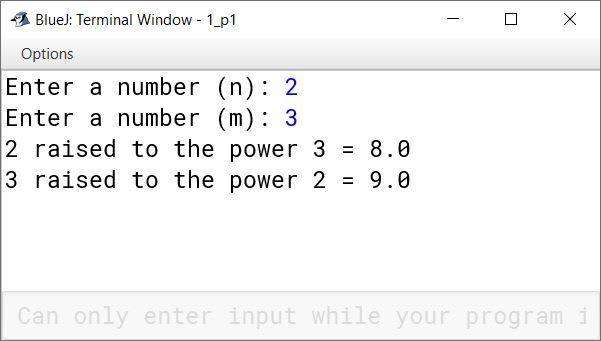#### Question 2

Write a program in Java to input perpendicular and base of a right angle triangle using the Scanner class. Calculate and display its hypotenuse using the formula given below:
h = √(p2 + b2)

```import java.util.Scanner;

public class KboatHypotenuse
{
public static void main(String args[]) {
Scanner in = new Scanner(System.in);
System.out.print("Enter Perpendicular: ");
double p = in.nextDouble();
System.out.print("Enter Base: ");
double b = in.nextDouble();

double h = Math.sqrt(Math.pow(p, 2) + Math.pow(b, 2));

System.out.println("Hypotenuse = " + h);
}
}```
##### Output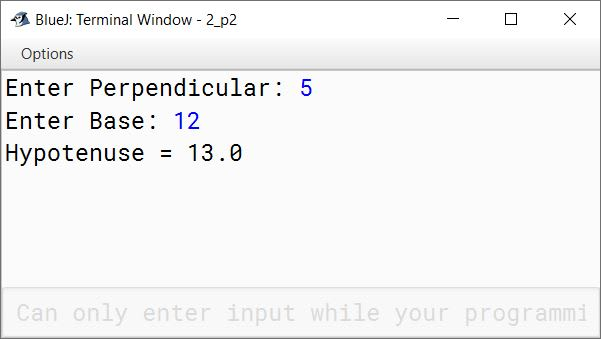#### Question 3

A shopkeeper offers 30% discount on purchasing articles, whereas the other shopkeeper offers two successive discounts 20% and 10% for purchasing the same articles. Write a program in Java to compute and display which is a better offer for a customer. Take the price of an article as the input using the Scanner class.

```import java.util.Scanner;

public class KboatDiscounts
{
public static void main(String args[]) {
Scanner in = new Scanner(System.in);
System.out.print("Enter price of article: ");
double price = in.nextDouble();

double d1 = price * 30 / 100.0;
double amt1 = price - d1;
System.out.println("30% discount = " + d1);
System.out.println("Amount after 30% discount = " + amt1);

double d2 = price * 20 / 100.0;
double amt2 = price - d2;
double d3 = amt2 * 10 / 100.0;
amt2 -= d3;
System.out.println("20% discount = " + d2);
System.out.println("10% discount = " + d3);
System.out.println("Amount after successive discounts = " + amt2);

if (amt1 < amt2) {
System.out.println("30% discount is a better offer");
}
else if (amt2 < amt1) {
System.out.println("Successive discounts are a better offer");
}
else {
System.out.println("Both offers are equal");
}
}
}```
##### Output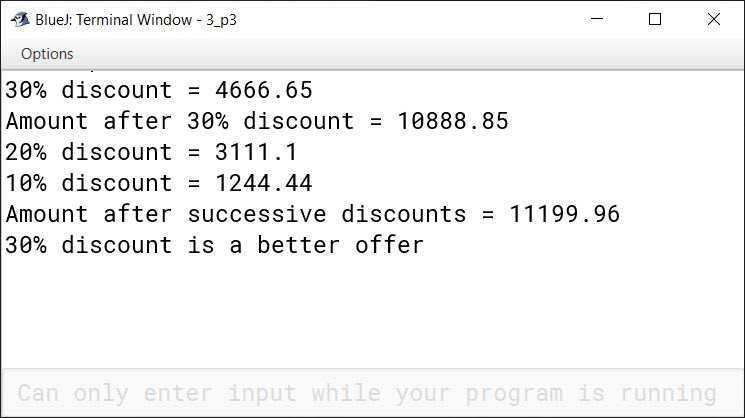#### Question 4

Write a program in Java to accept the cost price and the selling price of an article using the Scanner class. Check whether it incurs a profit or a loss. If profit happens, then find and display profit percent, else display loss percent.

[Hint: Profit percent = (Profit/cp)*100, Loss percent = (Loss/cp)*100]

```import java.util.Scanner;

public class KboatProfit
{
public static void main(String args[]) {
Scanner in = new Scanner(System.in);
System.out.print("Enter cost price of the article: ");
double cp = in.nextDouble();
System.out.print("Enter selling price of the article: ");
double sp = in.nextDouble();
double pl = sp - cp;
double percent = Math.abs(pl) / cp * 100;
if (pl > 0) {
System.out.println("Profit = " + pl);
System.out.println("Profit % = " + percent);
}
else if (pl < 0) {
System.out.println("Loss = " + Math.abs(pl));
System.out.println("Loss % = " + percent);
}
else {
System.out.println("Neither profit nor loss");
}
}
}```
##### Output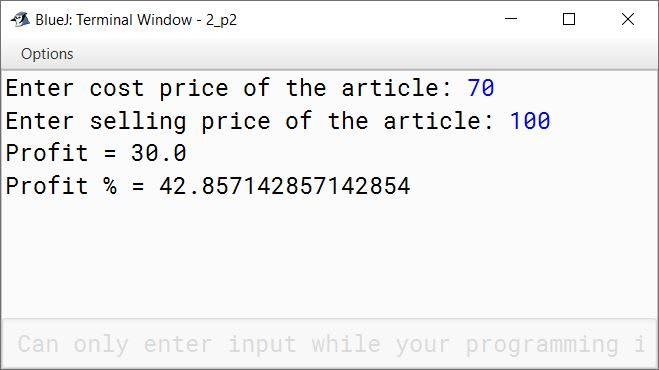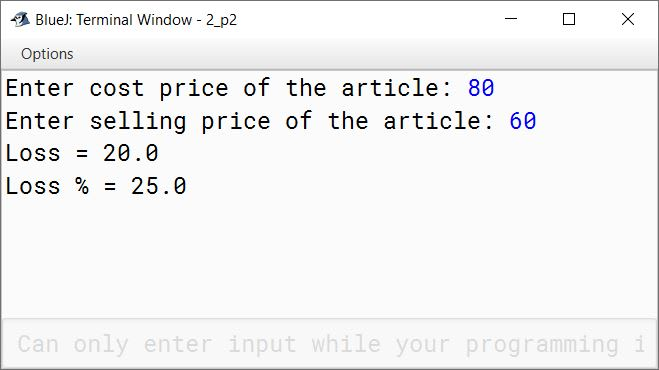#### Question 5

A triangle is said to be an 'Equable Triangle' if the area of the triangle is equal to its perimeter. Write a program to enter three sides of a triangle using the Scanner class. Check and display whether the triangle is equable or not.
For example, a right angled triangle with perpendicular, base and height as 5, 12 and 13 respectively has its area and perimeter same as 30.

```import java.util.Scanner;

public class KboatEquableTriangle
{
public static void main(String args[]) {

Scanner in = new Scanner(System.in);

System.out.println("Please enter the 3 sides of the triangle.");

System.out.print("Enter the first side: ");
double a = in.nextDouble();

System.out.print("Enter the second side: ");
double b = in.nextDouble();

System.out.print("Enter the third side: ");
double c = in.nextDouble();

double p = a + b + c;
double s = p / 2;
double area = Math.sqrt(s * (s - a) * (s - b) * (s - c));

if (area == p)
System.out.println("Entered triangle is equable.");
else
System.out.println("Entered triangle is not equable.");
}
}```
##### Output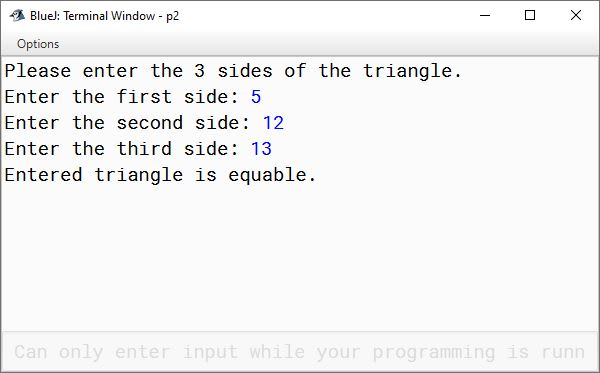#### Question 6

A special two-digit number is such that when the sum of its digits is added to the product of its digits, the result is equal to the original two-digit number.
Write a program to accept a two-digit number using the Scanner class. Check and display whether it is a special two-digit number or not.
Sample Input: 59
Sum of digits = 5 + 9 = 14
Product of digits = 5 * 9 = 45
Total of the sum of digits and product of digits = 14 + 45 = 59 .
Hence, 59 is a special two digit number.

```import java.util.*;

public class KboatSpecialNumber
{
public static void main(String args[]) {
Scanner in = new Scanner(System.in);

System.out.print("Enter a 2 digit number: ");
int num = in.nextInt();

int d1 = num % 10;
int d2 = num / 10;

int digitSum = d1 + d2;
int digitProduct  = d1 * d2;
int sum = digitSum + digitProduct;

if (sum == num) {
System.out.println(num + " is a Special 2-digit number");
}
else {
System.out.println(num + " is not a Special 2-digit number");
}

}
}```
##### Output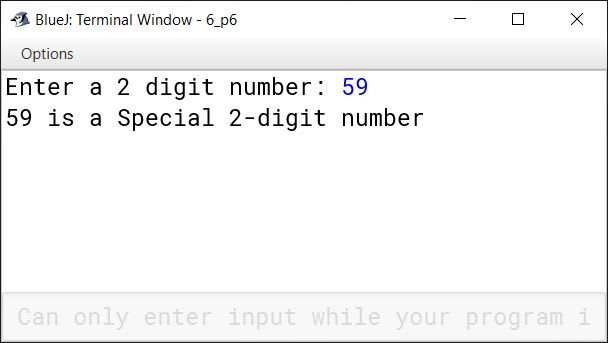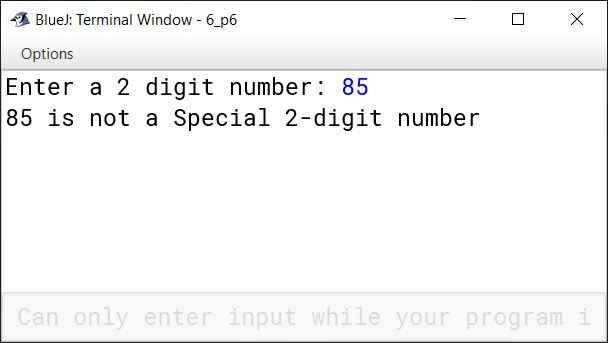#### Question 7

A library charges a fine for returning a book late after the due date as per the conditions given below:

No. of daysFine
First five days₹ 2.00 per day
Six to ten days₹ 5.00 per day
Above ten days₹ 10.00 per day

Design a program in Java assuming that a book is returned N days late. Input the value of N using the Scanner class. Calculate and display the fine to be paid to the library.

```import java.util.*;

public class KboatLibraryFine
{
public static void main(String args[])
{
Scanner in = new Scanner(System.in);

System.out.print("Enter the number of days : ");
int n = in.nextInt();

double fine = 0.0;

if (n <= 5)
fine = n * 2.0 ;
else if (n <= 10)
fine = 10 + (n - 5) * 5.0 ;
else
fine = 10 + 25 + (n - 10) * 10 ;

System.out.println("Fine to be paid = ₹" + fine);
}
}```
##### Output